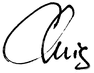# teaching machines

## CS 330 Lecture 27 – Fold

April 12, 2017 by . Filed under cs330, lectures, spring 2017.

Dear students,

Let’s start with a Program This! Complete two of the following functions based on your row number. All of them accept a list. Solve them using the pattern-matching style, with base cases and general cases defined separately. All of them should be two-liners.

1. upped, which generates an uppercase version of the incoming String (use toUpper :: Char -> Char)
2. any, which yields true if any of the boolean elements of the list is true, and false otherwise
3. product, which yields the product of all the elements in the list
4. size, which counts the number of elements in the list
5. join, which concatenates together all the elements of the list
6. twice, which generates a new list like the original, but with every element doubled
7. sum, which yields the sum of all the elements in the list
8. all, which yields true if all of the boolean elements of the list are true, and false otherwise

You will share your solutions up on the markerboard. From there we will abstract.

What do these solutions all have in common? Well, they all turn a list into some other summary value, whether that summary value is a representative number of another list. How do they different?

1. In their “default” or “zero” value that is invoked in the base case.
2. In their mixing or combining function, which stirs one of the elements into the summary value.

The abstraction of this algorithm is called fold, and the zero value and the mixing function will be the holes that we leave open. It’s interface will be something like this:

fold mix zero list

Let’s define it, postponing the type signature for a moment:

fold mix zero [] = zero
fold mix zero (first:rest) = mix first (fold mix zero rest)

We can apply a few syntactic niceties:

fold _ zero [] = zero
fold mix zero (first:rest) = mix first \$ fold mix zero rest

Now, let’s rewrite all of our functions from above using fold:

upped = fold (\c rest -> toUpper c : rest) []
any' = fold (||) False
product' = fold (*) 1
size = fold (\_ count -> 1 + count) 0
join = fold (++) ""
twice = fold (\x rest -> 2 * x : rest) []
sum' = fold (+) 0
all' = fold (&&) True

This rounds out our discussion of the big functional patterns. They should feel like adding SQL queries to our programming languages, ‘cuz we are!

Sincerely,